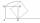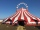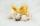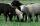# Z9–I–1

In all nine fields of given shape to be filled natural numbers so that:

• each of the numbers 2, 4, 6 and 8 is used at least once,
• four of the inner square boxes containing the products of the numbers of adjacent cells of the outer square,
• in the circle is the sum of the numbers of adjacent cells of the inner square.

Find out what the smallest and the largest number that can be written in a circle.

Result

a=##:  0
b=##:  0

Leave us a comment of example and its solution (i.e. if it is still somewhat unclear...):

Showing 0 comments:Be the first to comment!## Next similar examples:

1. AP - simpleDetermine the first nine elements of sequence if a10 = -1 and d = 4
2. Mrak - cloudIt is given segment AB of length 12 cm, where one side of the square MRAK laid on it. MRAK's side length 2 cm shown. MRAK gradually flips along the line segment AB the point R leaves a paper trail. Draw the whole track of point R until square can do the.
3. CandiesIn the box are 12 candies that look the same. Three of them are filled with nougat, five by nuts, four by cream. At least how many candies must Ivan choose to satisfy itself that the selection of two with the same filling? ?
4. Last digitWhat is the last number of 2016 power of 2017
5. Basket of fruitIn six baskets, the seller has fruit. In individual baskets, there are only apples or just pears with the following number of fruits: 5,6,12,14,23 and 29. "If I sell this basket," the salesman thinks, "then I will have just as many apples as a pear." Which
6. TicketsTickets to the zoo cost \$4 for children, \$5 for teenagers and \$6 for adults. In the high season, 1200 people come to the zoo every day. On a certain day, the total revenue at the zoo was \$5300. For every 3 teenagers, 8 children went to the zoo. How many te
7. CircusOn the circus performance was 150 people. Men were 10 less than women and children 50 more than adults. How many children were in the circus?
8. Sheep and cowsThere are only sheep and cows on the farm. Sheep is eight more than cows. The number of cows is half the number of sheep. How many animals live on the farm?
9. Find the sumFind the sum of all natural numbers from 1 and 100, which are divisible by 2 or 5
10. Theorem proveWe want to prove the sentense: If the natural number n is divisible by six, then n is divisible by three. From what assumption we started?
11. TunnelsMice had built an underground house consisting of chambers and tunnels: • each tunnel leading from the chamber to the chamber (none is blind) • from each chamber lead just three tunnels into three distinct chambers, • from each chamber mice can get to any
12. ExaminationThe class is 21 students. How many ways can choose two to examination?
13. BlocksThere are 9 interactive basic building blocks of an organization. How many two-blocks combinations are there?
14. ChordsHow many 4-tones chords (chord = at the same time sounding different tones) is possible to play within 7 tones?
15. Lord RamWhen lord Ram founded the breed white sheep was 8 more than black. Currently white sheep are four times higher than at the beginning and black three times more than at the beginning. White sheep is now 42 more than the black. How many white and black sh
16. Elimination methodSolve system of linear equations by elimination method: 5/2x + 3/5y= 4/15 1/2x + 2/5y= 2/15
17. Three unknownsSolve the system of linear equations with three unknowns: A + B + C = 14 B - A - C = 4 2A - B + C = 0